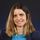cancel
Showing results for
Did you mean:Regular Visitor

## How to replace decimal values but retain the original whole number

I'm working with a netsuit timesheeting export that records a half fraction as .3

e.g. 37.5 hours a week shows as 37.3.
I have tried useing a conditional column to convert the .3 to a .5 but if the text contains .3 (else return the column value) however I can't figure out how to return the value before the decimal pointAm I using the correct method?

1 ACCEPTED SOLUTIONSuper User

Hi @Rotcorp

use the "Replace Value" option then manually edit thecode adding a conditional if statement and that says something like

each if Text.Contains ( [Column], ".3" ) then ".3" else null

3 REPLIES 3Resolver I

Hi. My suggestion is

1. Create a column that round down the value (column1) : Number.RoundDown([Total Hours])

2. Create a column2 that returns the decimal part, i.e..  [Column1] - [Total Hours]

3. Create column3 a which is [Column1]+0.5

4. Then make a conditional column

IF Column2 =0.7 than Column3

ELSE

[Total Hours]Super User

Hi @Rotcorp

use the "Replace Value" option then manually edit thecode adding a conditional if statement and that says something like

each if Text.Contains ( [Column], ".3" ) then ".3" else nullRegular Visitor

Thank you that worked.